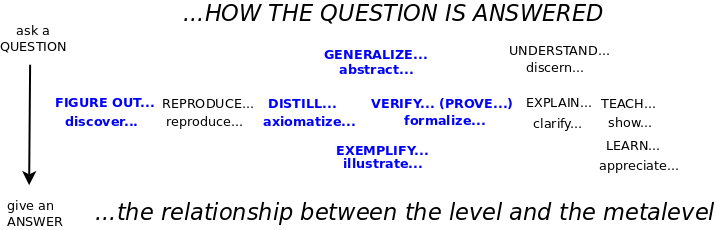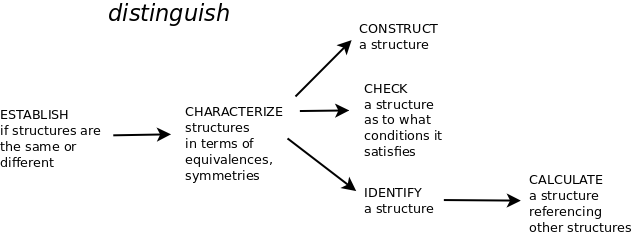• ms@ms.lt
• +370 607 27 665
• My work is in the Public Domain for all to share freely.

Introduction E9F5FC

Understandable FFFFFF

Questions FFFFC0

Notes EEEEEE

Software

See: Math Big Picture

Understand mathematics by describing it as an activity.

Consider math as the most general discipline for figuring things out, for relating questions and answers, in the spirit of games of understanding. Look for the role of duality and deviation from it.

Study the most basic math, what is taught in grade school. Study geometries as theories.

Given a theory, as in geometry, collect and study the questions that arise.

What kinds of mathematical activity are there, how are they related and what do they involve?

• Consider how all aspects of math may be generated.
• Relate that to the ways of figuring things out, and in particular, the three-cycle.A math result is an Alexandrian pattern which brings together:

• the forces in tension: the axioms or principles that it involves
• the instances, particular and general: the objects (of whatever degree of abstraction) that it holds for
• the logic that holds it together, the rule of thumb: an argumentation (a proof or demonstration)
• the way of figuring things out that brought it together

Counting: characterizing a set.

Note: the action becomes an object in its own right. For example, an object is characterized by whether it is an instance of itself or is not. The multiset of instances is then characterized by distinguishing a set of distinct instances. A set is characterized by counting it. But the count is not a set but a list. In this way, algebra unfolds to yield counting.

Study polymorphism of principles of basic math

Math should derive from logic, from perfect duality, and variation from it. The relation between logic and metalogic is given by our interpretation of a variable with reference to one of four levels. This establishes an analogy between logic, set theory, category theory and...? See logic.

List basic principles of math:

• Every answer is an amount and a unit. These are the grounds for recognizing differences (amounts) and similarities (units). "Comparing apples and oranges."
• Combine like units.
• List unlike units.
• Extend the domain.
• Every right triangle is half a rectangle.
• Every triangle is two right triangles.

#### MathActivity

Naujausi pakeitimai

 Puslapis paskutinį kartą pakeistas 2019 kovo 03 d., 22:08